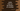# Kotlin program to find out the factors of a number# Kotlin program to find out the factors of a number :

In this tutorial, we will learn how to find out the factors of a number in Kotlin. We will show you two different ways : using a for loop and by using a while loop to solve this problem. The programs will print out the factors of a given number one by one. Let’s have a look :

## Find out the factors of a number using for loop in Kotlin :

First of all, let me show you how to find the factors list using a for loop in Kotlin :

``````fun main(args: Array) {
val no = 56

print("Factors : ")
for (i in 1..no) {
if (no % i == 0) {
print("\$i ")
}
}
}
``````

If you run the above program, it will print the below output :

``````Factors : 1 2 4 7 8 14 28 56
``````

### Explanation :

As you can see that we have used one for loop in this program. The loop run from 1 to the number. For each iteration, we have checked if the given number is divisible by current for loop counter or not. If yes, then we are printing out the result.

## Find out the factors of a number using while loop in Kotlin :

Now, let’s try to modify the above program with a while loop :

``````fun main(args: Array) {
val no = 56
var i = 1

print("Factors : ")
while (i <= no) {
if (no % i == 0) {
print("\$i ")
}
i++
}
}
``````

This program will print the same output as above. The only difference is that in the previous program, we have one for loop from i=1 to i=no and in this program, we have one while loop that will run till i <= no with initial value of i as 1. Logically, both of these programs are same, only syntax is different.

### Conclusion :

The main intension of this tutorial was to introduce you with while and for loops. Try to modify and run these examples and drop one comment below if you have any questions.

You might also like :